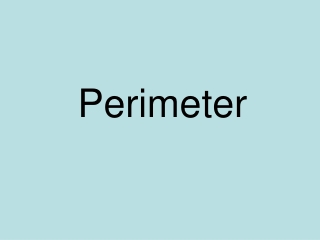DownloadDownload PresentationPerimeter

# Perimeter

Télécharger la présentation## Perimeter

- - - - - - - - - - - - - - - - - - - - - - - - - - - E N D - - - - - - - - - - - - - - - - - - - - - - - - - - -
##### Presentation Transcript

1. Perimeter

2. To find the perimeter we need to add all the measurements from around the outside. 8cm 8cm 8cm 8cm 8cm + 8cm + 8cm + 8cm = 32cm

3. 4cm 4cm Do we have to measure every side to work out the perimeter? 5cm 2cm 2cm 5cm 4cm

4. Write down the perimeter for each shape. Which shapes have the same perimeter? c a b e d

5. Write down the perimeter for each shape. Which shapes have the same perimeter? c a b Perimeter = 12cm Perimeter = 8cm e Perimeter = 12cm Perimeter = 22cm d Perimeter = 14cm